# IB DP Physics: HL复习笔记12.2.3 Deviations from Rutherford Scattering

### Deviations from Rutherford Scattering

• In the Rutherford scattering experiment, alpha particles are fired at a thin gold foil
• Some of the alpha particles are found to come straight back from the gold foil
• This indicates that there is electrostatic repulsion between the alpha particles and the gold nucleus
• At the point of closest approach, r, the repulsive force reduces the speed of the alpha particles to zero momentarily
• At this point, the initial kinetic energy of an alpha particle, Ek, is equal to electric potential energy, Ep
• Then the alpha particle will be electrostatically repelled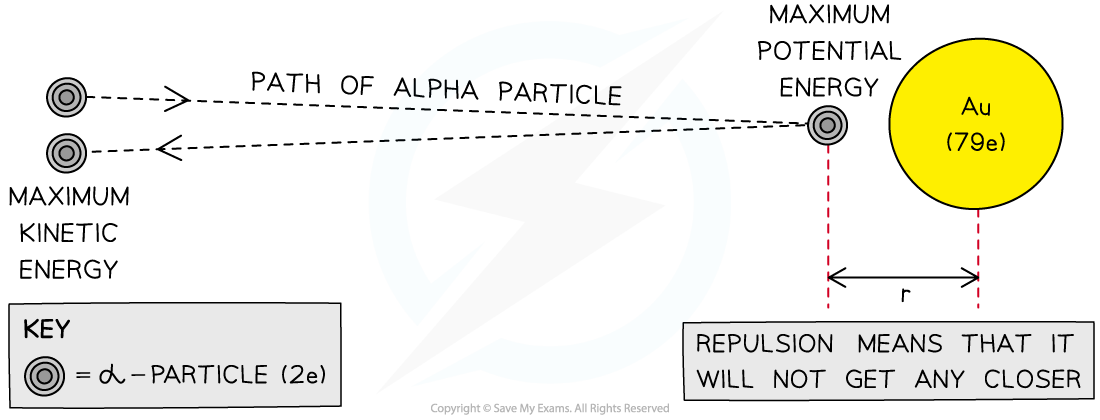The closest approach method of determining the size of a gold nucleus

• This experiment assumes that the alpha particles are only interacting through electrostatic repulsion

#### Deviations from Rutherford Scattering

• If the energy of the alpha particles exceeds 27.5 - 28 MeV, then they will be close enough to interact with the nucleus via the strong nuclear force
• The Rutherford formula describes this as it states that as the angle of scattering angle increases, the number of alpha particles scattered at that angle sharply decreases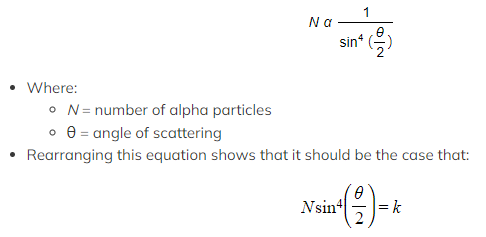• Experimental data bears this out, suggesting that the Rutherford formula is correct
• A number of assumptions are made in deriving this formula, with the main one being that the only force we need to consider is the electric force
• Deviations from Rutherford scattering are evidence of the strong nuclear force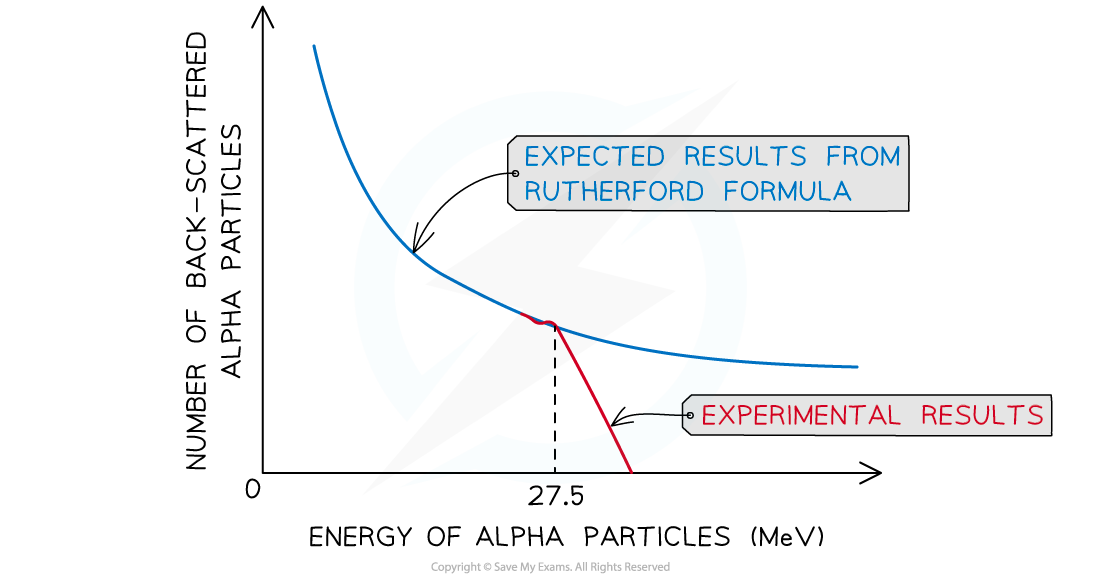The observed back-scattering from alpha particles strongly deviates from the predicted relationship based only on electromagnetic repulsion at 27.5 MeV

#### Worked Example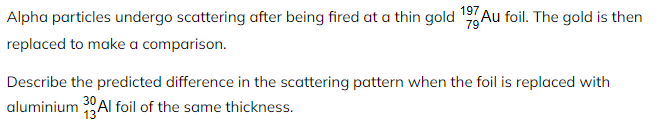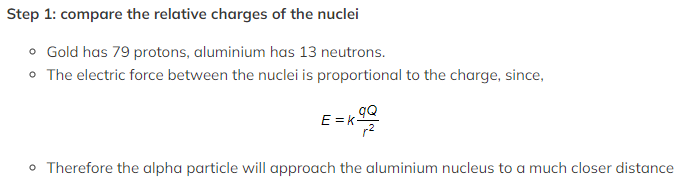Step 2: consider what causes deviation from Rutherford scattering

• Deviations from Rutherford scattering occur when forces apart from the electric force between the nuclei are at play
• At much smaller distances the effect of the strong nuclear force will be felt

Step 3: deduce the solution

• The alpha particles gets closer to the aluminium nucleus, and the strong nuclear force affects them
• More deviation will be seen with aluminium foil than with gold foil

#### Exam Tip

• The greatest deviations from Rutherford scattering occur when the energy of the alpha particles is high and the radius of the target nuclei is small (meaning it has a small nucleon number)